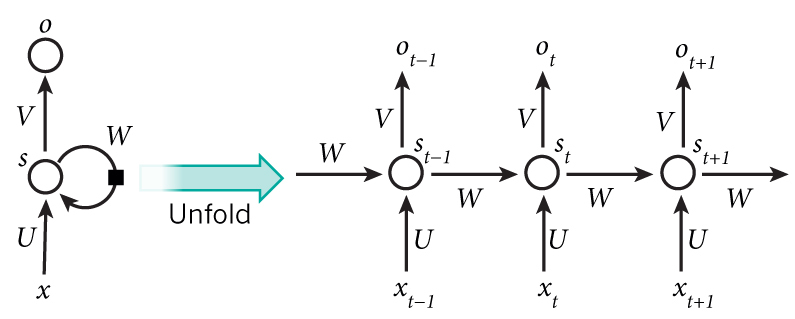语言模型

\$\$P(w_1,cdots,w_m) = prod_{i=1}^m P(w_i mid w_1,cdots,w_{i-1})\$\$

• 可以用于机器翻译或者语音识别中的正确句子打分
• 以概率生成新的句子

预处理并训练数据

4.建立训练数据的矩阵

RNN的输入和输出都是向量而不是字符串，我们需要把词与向量一一对应，通过index_to_wordword_to_index。比如一个训练的例子\$\$x\$\$为[0, 179, 341, 416]（注意到其中每个元素都是长度为vocabulary_size的one-hot向量，所以\$\$x\$\$实际上是一个矩阵），那么其label-\$\$y\$\$为[179, 341, 416, 1]，注意到我们的目标是预测下一个词，所以\$\$y\$\$就是\$\$x\$\$移动一位，并添加上最后的一个元素（预测词）的结果，其中SENTENCE_STARTSENTENCE_END分别为0和1.

##建立RNNRNN中的等式：
\$\$
s_t = tanh(Ux_t + Ws_{t-1})
o_t = softmax(Vs_t)
\$\$

\$\$
x_t in Bbb{R}^{8000} o_t in Bbb{R}^{8000} s_t in Bbb{R}^{100} U_t in Bbb{R}^{100 times 8000} V_t in Bbb{R}^{8000 times 100} W_t in Bbb{R}^{100 times 100}
\$\$

###初始化

###前向计算

###计算损失

\$\$
L(y,o) = – frac{1}{N} sum_{n in N} y_n log o_n
\$\$

###通过SGD和BPTT（BACKPROPAGATION THROUGH TIME ）训练RNN

###测试梯度

\$\$
frac{partial L}{partial{theta}} approx lim_{h rightarrow0} frac{J(theta + h)-J(theta – h)}{2h}
\$\$